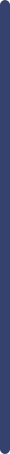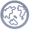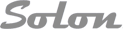新闻动态展会信息行业动态产品展示关于我们新闻中心产品展示联系我们> 首页 > 新闻中心
 Surface tensionSurface tension is a property of the surface of a liquid that allows it to resist an external force. It is revealed, for example, in floating of some objects on the surface of water, even though they are denser than water, and in the ability of some insects (e.g. water striders) and even reptiles (basilisk) to run on the water surface. This property is caused by cohesion of like molecules, and is responsible for many of the behaviors of liquids.

Surface tension has the dimension of force per unit length, or of energy per unit area. The two are equivalent—but when referring to energy per unit of area, people use the term surface energy—which is a more general term in the sense that it applies also to solids and not just liquids.

In materials science, surface tension is used for either surface stress or surface free energy.

[hide]

## Cause

The cohesive forces among the liquid molecules are responsible for this phenomenon of surface tension. In the bulk of the liquid, each molecule is pulled equally in every direction by neighboring liquid molecules, resulting in a net force of zero. The molecules at the surface do not have other molecules on all sides of them and therefore are pulled inwards. This creates some internal pressure and forces liquid surfaces to contract to the minimal area.

Surface tension is responsible for the shape of liquid droplets. Although easily deformed, droplets of water tend to be pulled into a spherical shape by the cohesive forces of the surface layer. In the absence of other forces, including gravity, drops of virtually all liquids would be perfectly spherical. The spherical shape minimizes the necessary "wall tension" of the surface layer according to Laplace's law.

Another way to view it is in terms of energy. A molecule in contact with a neighbor is in a lower state of energy than if it were alone (not in contact with a neighbor). The interior molecules have as many neighbors as they can possibly have, but the boundary molecules are missing neighbors (compared to interior molecules) and therefore have a higher energy. For the liquid to minimize its energy state, the number of higher energy boundary molecules must be minimized. The minimized quantity of boundary molecules results in a minimized surface area.

As a result of surface area minimization, a surface will assume the smoothest shape it can (mathematical proof that "smooth" shapes minimize surface area relies on use of the Euler–Lagrange equation). Since any curvature in the surface shape results in greater area, a higher energy will also result. Consequently the surface will push back against any curvature in much the same way as a ball pushed uphill will push back to minimize its gravitational potential energy.

## Effects in everyday life

### Water

Several effects of surface tension can be seen with ordinary water:

A. Beading of rain water on the surface of a waxy surface, such as an automobile. Water adheres weakly to wax and strongly to itself, so water clusters into drops. Surface tension gives them their near-spherical shape, because a sphere has the smallest possible surface area to volume ratio.

B. Formation of drops occurs when a mass of liquid is stretched. The animation shows water adhering to the faucet gaining mass until it is stretched to a point where the surface tension can no longer bind it to the faucet. It then separates and surface tension forms the drop into a sphere. If a stream of water were running from the faucet, the stream would break up into drops during its fall. Gravity stretches the stream, then surface tension pinches it into spheres.

C. Flotation of objects denser than water occurs when the object is nonwettable and its weight is small enough to be borne by the forces arising from surface tension. For example, water striders use surface tension to walk on the surface of a pond. The surface of the water behaves like an elastic film: the insect's feet cause indentations in the water's surface, increasing its surface area.

D. Separation of oil and water is caused by a tension in the surface between dissimilar liquids. This type of surface tension is called "interface tension", but its physics are the same.

E. Tears of wine is the formation of drops and rivulets on the side of a glass containing an alcoholic beverage. Its cause is a complex interaction between the differing surface tensions of water and ethanol; it is induced by a combination of surface tension modification of water by ethanol together with ethanol evaporating faster than water.

### Surfactants

Surface tension is visible in other common phenomena, especially when surfactants are used to decrease it:

• Soap bubbles have very large surface areas with very little mass. Bubbles in pure water are unstable. The addition of surfactants, however, can have a stabilizing effect on the bubbles (see Marangoni effect). Notice that surfactants actually reduce the surface tension of water by a factor of three or more.
• Emulsions are a type of solution in which surface tension plays a role. Tiny fragments of oil suspended in pure water will spontaneously assemble themselves into much larger masses. But the presence of a surfactant provides a decrease in surface tension, which permits stability of minute droplets of oil in the bulk of water (or vice versa).

## Basic physics

### Two definitionsDiagram shows, in cross-section, a needle floating on the surface of water. Its weight, Fw, depresses the surface, and is balanced by the surface tension forces on either side, Fs, which are each parallel to the water's surface at the points where it contacts the needle. Notice that the horizontal components of the two Fs arrows point in opposite directions, so they cancel each other, but the vertical components point in the same direction and therefore add up to balance Fw.

Surface tension, represented by the symbol γ is defined as the force along a line of unit length, where the force is parallel to the surface but perpendicular to the line. One way to picture this is to imagine a flat soap film bounded on one side by a taut thread of length, L. The thread will be pulled toward the interior of the film by a force equal to 2$\scriptstyle\gamma$L (the factor of 2 is because the soap film has two sides, hence two surfaces). Surface tension is therefore measured in forces per unit length. Its SI unit is newton per meter but the cgs unit of dyne per cm is also used. One dyn/cm corresponds to 0.001 N/m.

An equivalent definition, one that is useful in thermodynamics, is work done per unit area. As such, in order to increase the surface area of a mass of liquid by an amount, δA, a quantity of work,$\scriptstyle\gamma$δA, is needed. This work is stored as potential energy. Consequently surface tension can be also measured in SI system as joules per square meter and in the cgs system as ergs per cm2. Since mechanical systems try to find a state of minimum potential energy, a free droplet of liquid naturally assumes a spherical shape, which has the minimum surface area for a given volume.

The equivalence of measurement of energy per unit area to force per unit length can be proven by dimensional analysis.

### Surface curvature and pressureSurface tension forces acting on a tiny (differential) patch of surface. δθx and δθy indicate the amount of bend over the dimensions of the patch. Balancing the tension forces with pressure leads to the Young–Laplace equation

If no force acts normal to a tensioned surface, the surface must remain flat. But if the pressure on one side of the surface differs from pressure on the other side, the pressure difference times surface area results in a normal force. In order for the surface tension forces to cancel the force due to pressure, the surface must be curved. The diagram shows how surface curvature of a tiny patch of surface leads to a net component of surface tension forces acting normal to the center of the patch. When all the forces are balanced, the resulting equation is known as the Young–Laplace equation:$\Delta p\ =\ \gamma \left( \frac{1}{R_x} + \frac{1}{R_y} \right)$

where:

• Δp is the pressure difference.
•$\scriptstyle\gamma$ is surface tension.
• Rx and Ry are radii of curvature in each of the axes that are parallel to the surface.

The quantity in parentheses on the right hand side is in fact (twice) the mean curvature of the surface (depending on normalisation).

Solutions to this equation determine the shape of water drops, puddles, menisci, soap bubbles, and all other shapes determined by surface tension (such as the shape of the impressions that a water strider's feet make on the surface of a pond).

The table below shows how the internal pressure of a water droplet increases with decreasing radius. For not very small drops the effect is subtle, but the pressure difference becomes enormous when the drop sizes approach the molecular size. (In the limit of a single molecule the concept becomes meaningless.)

Δp for water drops of different radii at STP
Droplet radius 1 mm 0.1 mm 1 μm 10 nm
Δp (atm) 0.0014 0.0144 1.436 143.6

### Liquid surface

To find the shape of the minimal surface bounded by some arbitrary shaped frame using strictly mathematical means can be a daunting task. Yet by fashioning the frame out of wire and dipping it in soap-solution, a locally minimal surface will appear in the resulting soap-film within seconds.

The reason for this is that the pressure difference across a fluid interface is proportional to the mean curvature, as seen in the Young-Laplace equation. For an open soap film, the pressure difference is zero, hence the mean curvature is zero, and minimal surfaces have the property of zero mean curvature.

### Contact angles

The surface of any liquid is an interface between that liquid and some other medium.[note 1] The top surface of a pond, for example, is an interface between the pond water and the air. Surface tension, then, is not a property of the liquid alone, but a property of the liquid's interface with another medium. If a liquid is in a container, then besides the liquid/air interface at its top surface, there is also an interface between the liquid and the walls of the container. The surface tension between the liquid and air is usually different (greater than) its surface tension with the walls of a container. And where the two surfaces meet, their geometry must be such that all forces balance.Forces at contact point shown for contact angle greater than 90° (left) and less than 90° (right)

Where the two surfaces meet, they form a contact angle,$\theta$, which is the angle the tangent to the surface makes with the solid surface. The diagram to the right shows two examples. Tension forces are shown for the liquid-air interface, the liquid-solid interface, and the solid-air interface. The example on the left is where the difference between the liquid-solid and solid-air surface tension,$\gamma_{\mathrm{ls}} - \gamma_{\mathrm{sa}}$, is less than the liquid-air surface tension,$\gamma_{\mathrm{la}}$, but is nevertheless positive, that is$\gamma_{\mathrm{la}}\ >\ \gamma_{\mathrm{ls}} - \gamma_{\mathrm{sa}}\ >\ 0$

In the diagram, both the vertical and horizontal forces must cancel exactly at the contact point. The horizontal component of$f_\mathrm{la}$ is canceled by the adhesive force,$f_\mathrm{A}$.$f_\mathrm{A}\ =\ f_\mathrm{la} \sin \theta$

The more telling balance of forces, though, is in the vertical direction. The vertical component of$f_\mathrm{la}$ must exactly cancel the force,$f_\mathrm{ls}$.$f_\mathrm{ls} - f_\mathrm{sa}\ =\ -f_\mathrm{la} \cos \theta$

Liquid Solid Contact angle
water
 soda-lime glass lead glass fused quartz
ethanol
diethyl ether
carbon tetrachloride
glycerol
acetic acid
water paraffin wax 107°
silver 90°
methyl iodide soda-lime glass 29°
lead glass 30°
fused quartz 33°
mercury soda-lime glass 140°
Some liquid-solid contact angles

Since the forces are in direct proportion to their respective surface tensions, we also have:$\gamma_\mathrm{ls} - \gamma_\mathrm{sa}\ =\ -\gamma_\mathrm{la} \cos \theta$

where

•$\gamma_\mathrm{ls}$ is the liquid-solid surface tension,
•$\gamma_\mathrm{la}$ is the liquid-air surface tension,
•$\gamma_\mathrm{sa}$ is the solid-air surface tension,
•$\theta$ is the contact angle, where a concave meniscus has contact angle less than 90° and a convex meniscus has contact angle of greater than 90°.

This means that although the difference between the liquid-solid and solid-air surface tension,$\gamma_\mathrm{ls} - \gamma_\mathrm{sa}$, is difficult to measure directly, it can be inferred from the easily measured contact angle,$\theta$, if the liquid-air surface tension,$\gamma_\mathrm{la}$, is known.

This same relationship exists in the diagram on the right. But in this case we see that because the contact angle is less than 90°, the liquid-solid/solid-air surface tension difference must be negative:$\gamma_\mathrm{la}\ >\ 0\ >\ \gamma_\mathrm{ls} - \gamma_\mathrm{sa}$

#### Special contact angles

Observe that in the special case of a water-silver interface where the contact angle is equal to 90°, the liquid-solid/solid-air surface tension difference is exactly zero.

Another special case is where the contact angle is exactly 180°. Water with specially prepared Teflon approaches this. Contact angle of 180° occurs when the liquid-solid surface tension is exactly equal to the liquid-air surface tension.$\gamma_{\mathrm{la}}\ =\ \gamma_{\mathrm{ls}} - \gamma_\mathrm{sa}\ >\ 0\qquad \theta\ =\ 180^\circ$首页 / 新闻中心 / 产品展示 / 技术文章 / 下载中心 / 关于我们 / 客户留言 / 人才招聘 / 联系我们 版权所有 2005-2015 上海梭伦信息科技有限公司 电话：021-51036075，400 0055117， 上海梭伦信息科技有限公司(www.solon17.com)主要提供：接触角测试仪 ,表面张力仪,界面张力仪,接触角测量仪,水滴角测试仪，水滴角测试仪，接触角测定仪，光学接触角测量仪 网站地图 站点地图